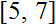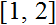# Volume 15, 2009, Number 4

Volume 15Number 1Number 2Number 3 ▷ Number 4

Note on path signed graphs
Original research paper. Pages 1—6
P. Siva Kota Reddy and M. S. Subramanya
Full paper (PDF, 129 Kb) | Abstract
Data in the social sciences can often modeled using signed graphs, graphs where every edge has a sign + or −, or marked graphs, graphs where every vertex has a sign + or −. The path graph Pk(G) of a graph G is obtained by representing the paths Pk in G by vertices whenever the corresponding paths Pk in G form a path Pk+1 or a cycle Ck. In this note, we introduce a natural extension of the notion of path graphs to the realm of signed graphs. It is shown that for any signed graph S, Pk(S) is balanced. The concept of a line signed graph is generalized to that of a path signed graphs. Further, in this note we discuss the structural characterization of path signed graphs. Also, we characterize signed graphs which are switching equivalent to their path signed graphs P3(S) (P4(S)).

On the linear systems over rhotrices
Original research paper. Pages 7—12
Full paper (PDF, 147 Kb) | Abstract

Let A, B and C be rhotrices. In this paper we investigate systems of the form AB = C and come up with conditions necessary for their solvability. We also outline a direct procedure for computing an n-th root of a rhotrix.

Structure and spectra of the components of primitive Pythagorean triples and Fermat’s last theorem
Original research paper. Pages 13—22
J. V. Leyendekkers, A. G. Shannon and C. K. Wong
Full paper (PDF, 204 Kb) | Abstract

Certain characteristics of Pythagorean triples are analysed using Integer Structure via the modular ring, Z4. The fact (as shown by Fermat’s Last Theorem) that all the components of a triple cannot simultaneously be an even power n (with ½n even) is illustrated via the spectra of the right-end-digits of the components.

A remark on an arithmetic function. Part 3
Original research paper. Pages 23—27
Krassimir Atanassov
Full paper (PDF, 126 Kb) | Abstract

In a series of papers the author studied some properties of the well-known arithmetic functions φ and σ (see, e.g.). With respect to these research, a new function was defined inand some of its properties were discussed there. Here we shall continue this research.

Volume 15Number 1Number 2Number 3 ▷ Number 4